# Trigonometry Formulas | sin θ, cos θ, tan θ, cosec θ, sec θ, cot θ | Trigonometry Basics

In this notes we'll cover topics about Trigonometry. We'll study about what is Trigonometry, why one should study Trigonometry and then about the basic formulae of Trigonometric Ratios.
Trigonometry is the study of triangles. More specifically right angled triangles.

## Why Trigonometry?

To understand why one should use Trigonometry, it is important to understand where you can use it or apply it. Let us imagine that you are visiting the Statue of Unity (world's tallest Statue, situated in Gujarat-India).

You want to know the height of this statue by standing at a distance from it without actually measuring the height of it. We can visualize a right angled triangle being formed by a ray of sight from your eye to the top of the statue (this forms the hypotenuse), the distance between you and the statue (as one side of the triangle) and the line representing the height of the statue (as the third side of the triangle). This visualization is shown in the image below.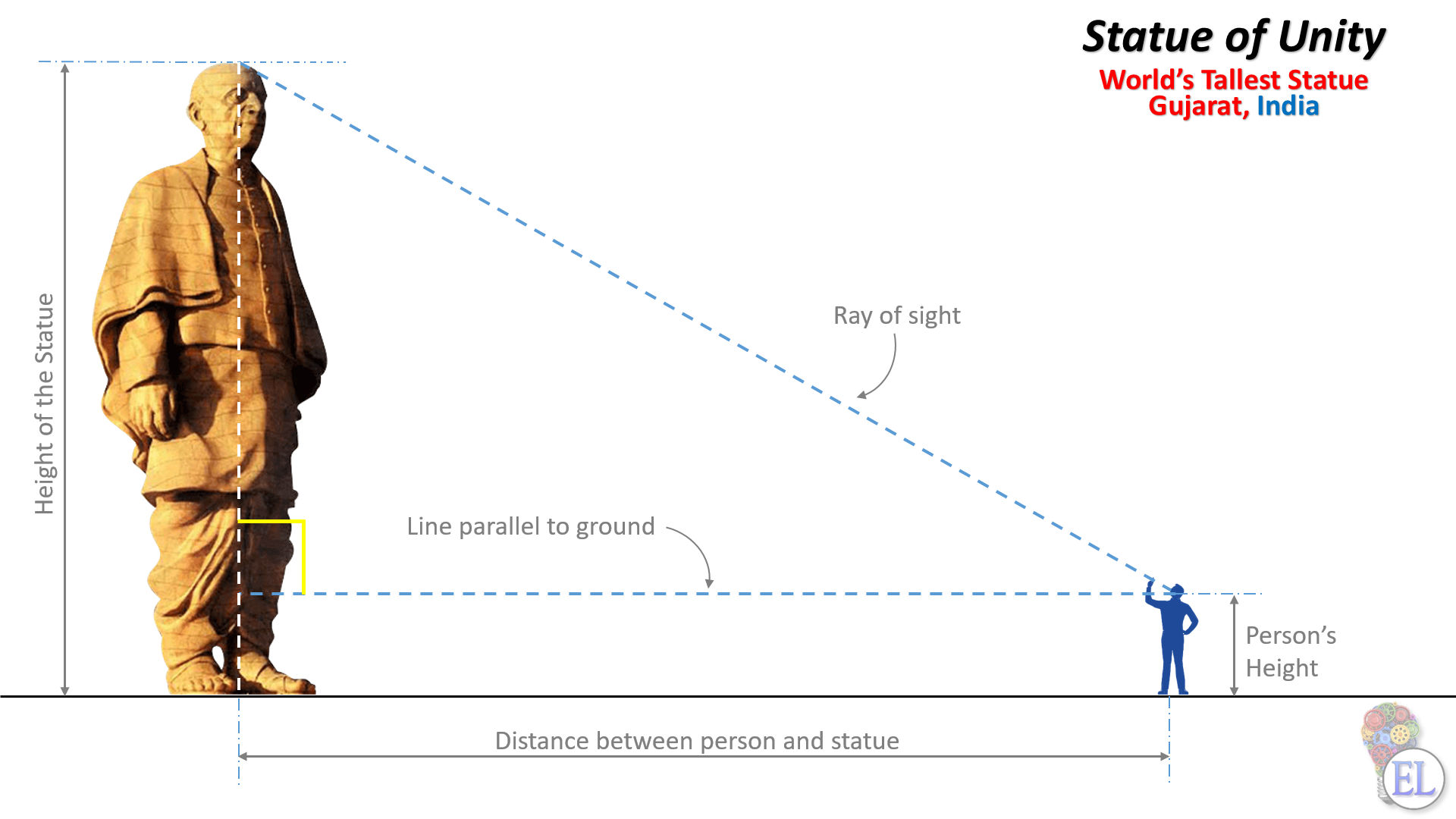Applying some basic trigonometry formulas it becomes easy to calculate the height of the statue.

## What is Trigonometry?

Trigonometry consists of three words - tri, gon and metron. Tri means three, gon means sides and metorn means measure. Hence we can say that Trigonometry is the study of relationships between the sides and angles of a triangle, more specifically right angle triangle.

## Trigonometry terminology

Consider a right angle triangle ABC, where $\angle B = 90 \degree$ as shown in the figure below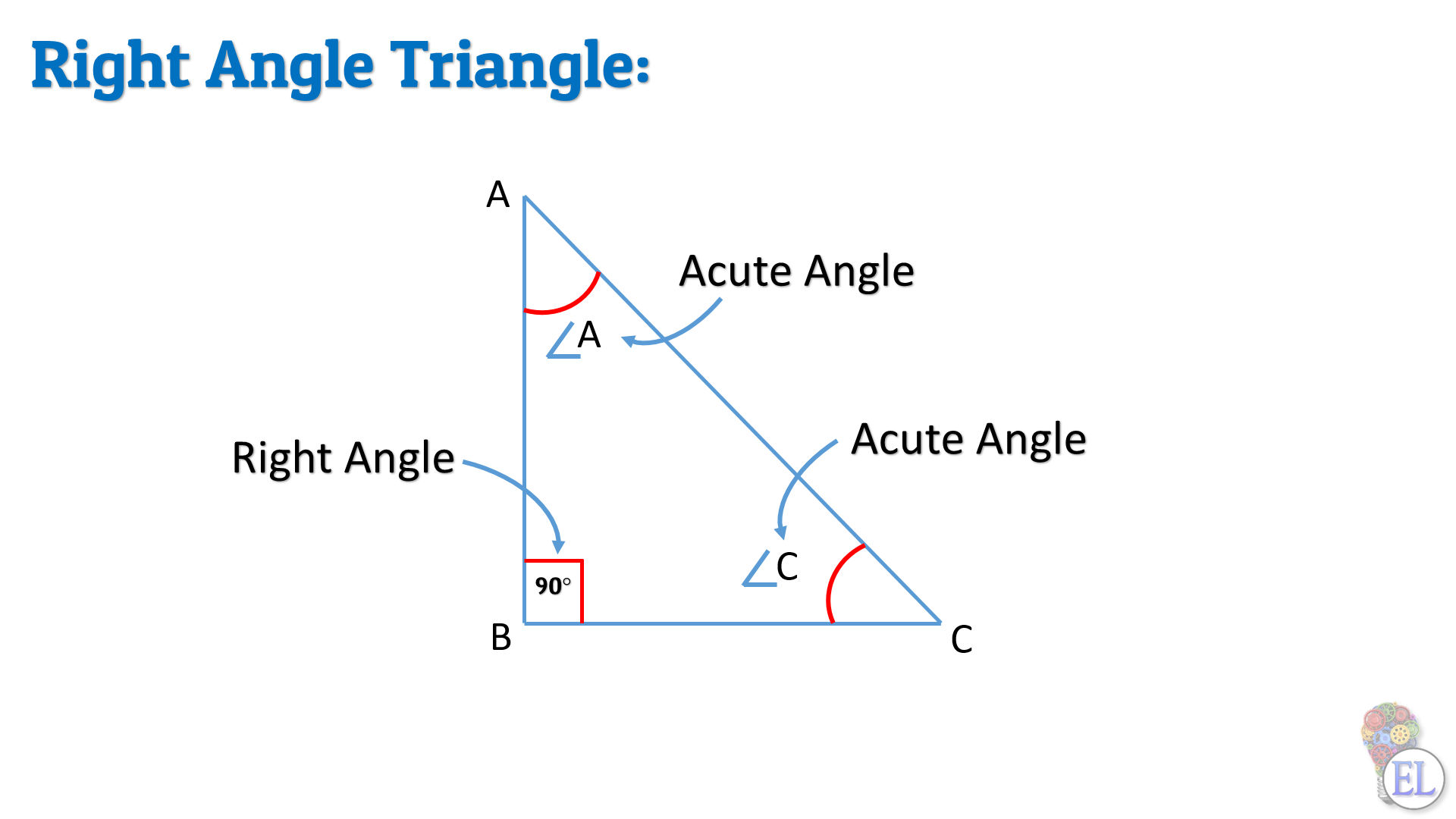### Proof: $\angle A$ and $\angle C$ are acute angle triangles

We know that the sum of all the angles in a triangle is $180 \degree$. Hence in our example of $\triangle ABC$ we can say that,

$\angle A + \angle B + \angle C = 180 \degree$

In $\triangle ABC$, $\angle B$ is $90 \degree$, so we can solve the above statement as:

\begin{aligned} \angle A + 90 \degree + \angle C &= 180 \degree \\ \angle A + \angle C &= 180 \degree - 90 \degree \\ \angle A + \angle C &= 90 \degree \\ \end{aligned}

Since the addition of $\angle A$ and $\angle B$ is equal to $90 \degree$. Both these angles are less than $90 \degree$. Therefore, $\angle A$ and $\angle C$ are acute angles.

### What are acute angles?

If the measure of an angle is less than $90 \degree$, that angle is known as an acute angle.

Let us start with $\angle C$, as shown in the figure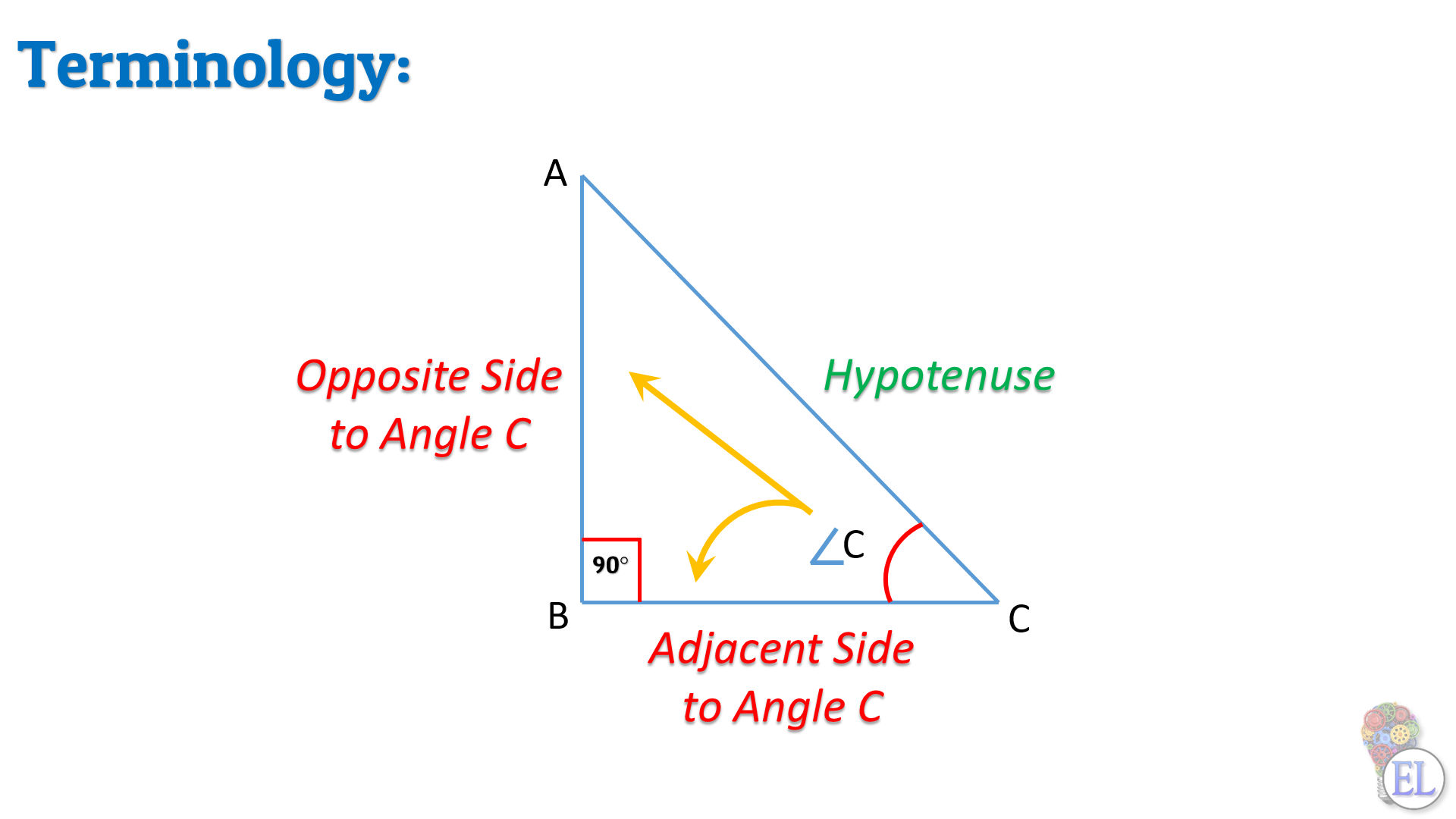\begin{aligned} \overline{AB} &= \text{Opposite side to } \angle C \text{ and} \\ \overline{BC} &= \text{Adjacent side to } \angle C \\ \overline{AC} &= \text{Hypotenuse of the right angle triangle} \end{aligned}

Remember: Hypotenuse is the longest side of a right angle triangle.

Similarly for $\angle A$, as shown in the figure

Remember: The terminology for Trigonometry changes with respect to angles.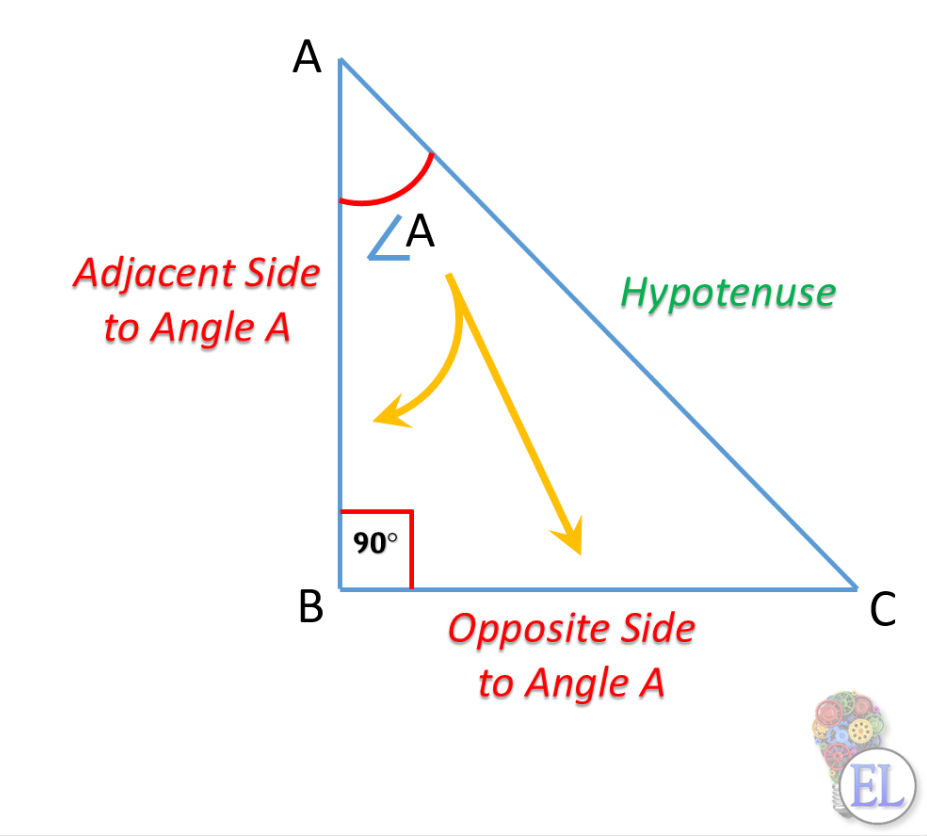\begin{aligned} \overline{BC} &= \text{Opposite side to } \angle A \text{ and} \\ \overline{AB} &= \text{Adjacent side to } \angle A \\ \overline{AC} &= \text{Hypotenuse of the right angle triangle} \\ & \quad \text{(hypotenuse will still remain the same)} \end{aligned}

## Trigonometric Ratios

Now let us learn about the different trigonometric ratios with their abbreviations. For explanation purpose let's consider these ratios with respect to $\angle A$

Ratios Abbreviations
sine of $\angle A$ $\sin A$
co-sine of $\angle A$ $\cos A$
tangent of $\angle A$ $\tan A$
co-tangent of $\angle A$ $\cot A$
secant of $\angle A$ $\sec A$
co-secant of $\angle A$ $\cosec A$

Note: "co" before the ratios stands for the Complementary of that specific ratio.## Deriving the values of sine and co-sine

Let us now derive the values of $\sin A$ and $\cos A$ based on the trigonometric ratios that we learned above

Remember:

Formulae for $\sin A$:

$\sin A = {\text {Opposite side of } \angle A \over Hypotenuse}$

Formulae for $\cos A$:

$\cos A = {\text {Adjacent side of } \angle A \over Hypotenuse}$

Using these formulas:

\begin{aligned} \sin A &= {\text {Opposite side of } \angle A \over Hypotenuse} \\ \\ &= {BC \over AC} \\ \\ \\ \cos A &= {\text {Adjacent side of } \angle A \over Hypotenuse} \\ \\ &= {AB \over AC} \\ \end{aligned}

Remember: $\sin$ and $\cos$ are fundamental trigonometric ratios and the others are derived ratios.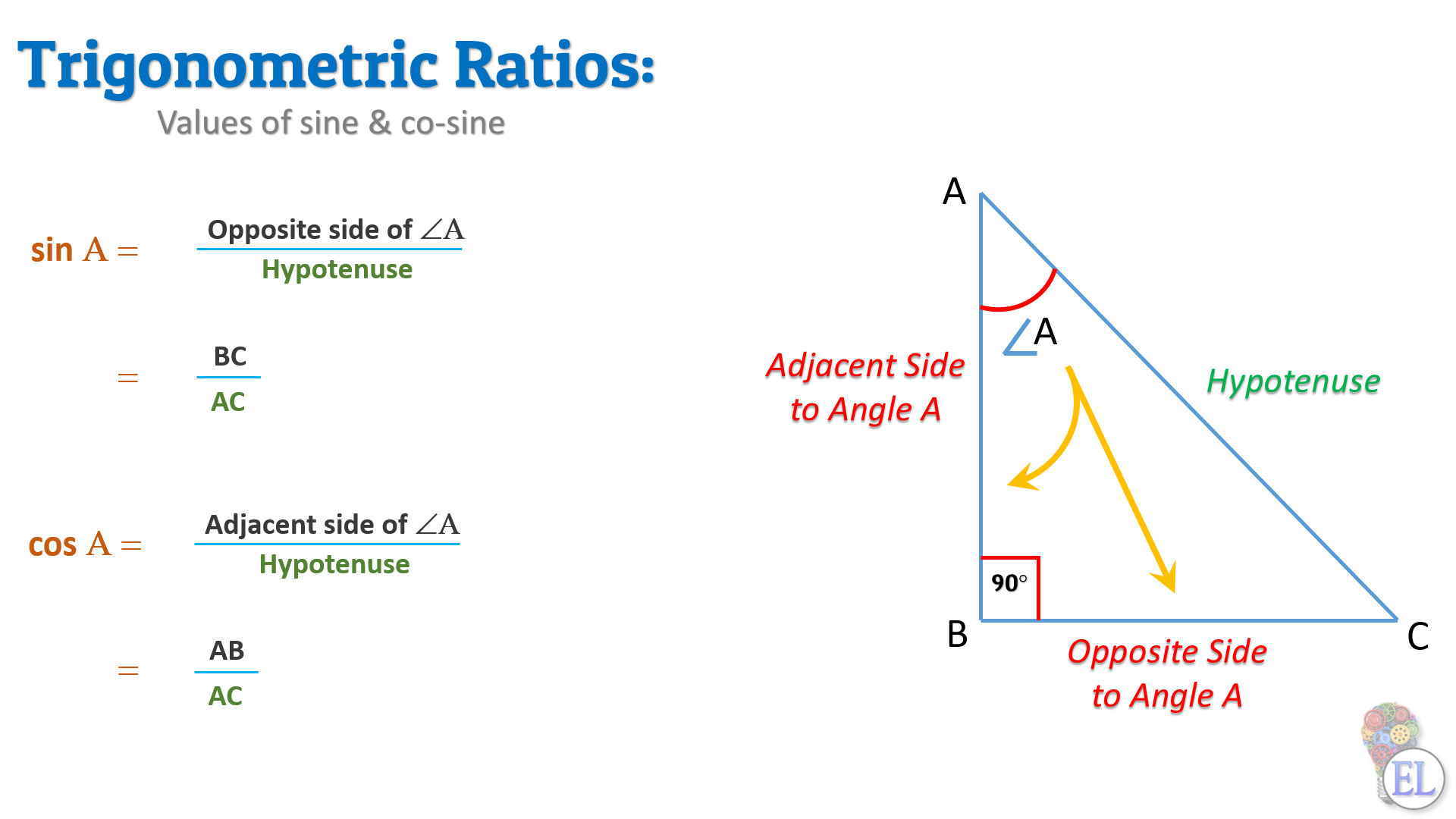We have now used all the sides of triangle ABC to derive the values of $\sin A$ and $\cos A$. In a similar manner, you can now derive the $\sin$ and $\cos$ for $\angle C$.

## Deriving the values of other trigonometric ratios from sine and co-sine

### Tangent of $\angle A$

\begin{aligned} \tan A &= {\sin A \over \cos A} \\ \\ &= {{\text{Opposite side of } \angle A \over hypotenuse} \over {\text{Adjacent side of } \angle A \over hypotenuse}} \\ \\ &= {{\text{Opposite side of } \angle A \over \cancel{hypotenuse}} \over {\text{Adjacent side of } \angle A \over \cancel{hypotenuse}}} \\ \\ \tan A &= {\text{Opposite side of } \angle A \over \text{Adjacent side of } \angle A} \\ \\ &= {BC \over AB} \end{aligned}

### Co-Tangent of $\angle A$

\begin{aligned} \cot A &= {\cos A \over \sin A} \\ \\ &= {{\text{Adjacent side of } \angle A \over \cancel{hypotenuse}} \over {\text{Opposite side of } \angle A \over \cancel{hypotenuse}}} \\ \\ \cot A &= {\text{Adjacent side of } \angle A \over \text{Opposite side of } \angle A} \\ \\ &= {AB \over BC} \end{aligned}

### Secant of $\angle A$

\begin{aligned} \sec A &= {1 \over \cos A} \\ &= {1 \over {\text {Adjacent side of } \angle A \over Hypotenuse}} \\ \\ \sec A &= {Hypotenuse \over \text {Adjacent side of } \angle A} \\ \\ &= {AC \over AB} \end{aligned}

### Co-Secant of $\angle A$

\begin{aligned} \cosec A &= {1 \over \sin A} \\ &= {1 \over {\text {Opposite side of } \angle A \over Hypotenuse}} \\ \\ \sec A &= {Hypotenuse \over \text {Opposite side of } \angle A} \\ \\ &= {AC \over BC} \end{aligned}

Since you now know how to calculate the trigonometric ratios, here's a tip for remembering these formulae:

1. We have $\sin$ and $\cos$ as the fundamental trigonometric ratios

2. If you know the formulae of $\sin$ and $\cos$ you can easily derive others

3. tan is the ratio of $\sin$ and $\cos$ and $\cot$ is the reciprocal of $\tan$

4. $\sec$ is the reciprocal of $\cos$

5. And $\cosec$ is the reciprocal of $\sin$